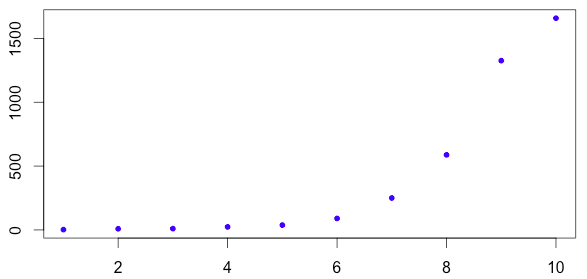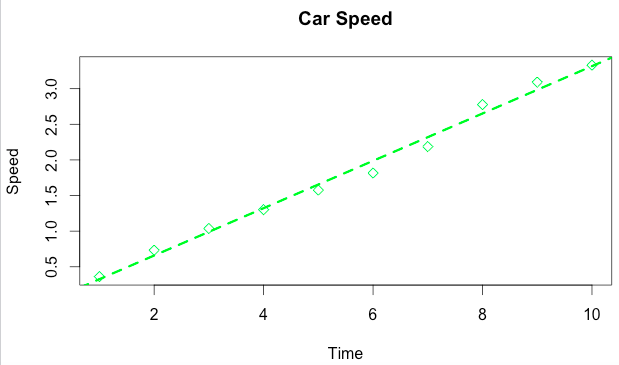1
2
3
4
5
#

R - Exponential Growth Modeling

Exponential Example A

The data set is x=(1, 2, 3, 4, 5, 6, 7, 8, 9, 10) and y=(2.4, 8.8, 9.9, 23.5, 38.1, 90.1, 250.1, 587.9, 1325.7, 1658.3)

Store the data under the variables "x" and "y"

x=c(1, 2, 3, 4, 5, 6, 7, 8, 9, 10)
y=c(2.4, 8.8, 9.9, 23.5, 38.1, 90.1, 250.1, 587.9, 1325.7, 1658.3)

If these points are plotted the exponential nature of the data is evident, as seen belowFor this exponential model take the log of the y values, and store it under a random variable, "ly"

ly=log10(y)

Plot the new graph, using the "x" and "ly" points

plot(x, ly,
col="purple",
pch=5,
xlab="Time",
ylab="Speed",
main="Car Speed")

Create the line of best fit for the exponential model, stored under the variable "model"

model=lm(ly~x)

Add the line to the graph

abline(model,
col="purple",
lty=3,
lwd=2)

The final code is below

x=c(1, 2, 3, 4, 5, 6, 7, 8, 9, 10)
y=c(2.4, 8.8, 9.9, 23.5, 38.1, 90.1, 250.1, 587.9, 1325.7, 1658.3)
ly=log10(y)
plot(x, ly, col="purple", pch=5, xlab="Time", ylab="Speed", main="Car Speed")
model=lm(ly~x)
abline(model, col="purple", lty=3, lwd=2)

This is the new graphExponential Example B

The data set is x=(1, 2, 3, 4, 5, 6, 7, 8, 9, 10) and y=(2.3, 5.4, 10.9, 20.0, 37.7, 65.4, 153.8, 597.4, 1236.2, 2137.0)

Store the data under the variables "x" and "y"

x=c(1, 2, 3, 4, 5, 6, 7, 8, 9, 10)
y=c(2.3, 5.4, 10.9, 20.0, 37.7, 65.4, 153.8, 597.4, 1236.2, 2137.0)

If these points are plotted the exponential nature of the data is evident, as seen belowFor an exponential model take the log of the y values, and store it under a random variable, here "lgy"

lgy=log10(y)

Plot the new graph, using the "x" and "ly" points

plot(x, lgy,
main="Car Speed",
xlab="Time",
ylab="Speed",
pch=5,
col="green2")

Create the line of best fit for the exponential model, stored under the variable "tr"

tr=lm(ly~x)


Add the line to the graph

abline(tr,
col="green2",
lwd=3,
lty=2)

The final code is below

x=c(1, 2, 3, 4, 5, 6, 7, 8, 9, 10)
y=c(2.3, 5.4, 10.9, 20.0, 37.7, 65.4, 153.8, 597.4, 1236.2, 2137.0)
lgy=log10(y)
plot(x, lgy, main="Car Speed", pch=5, xlab="Time", ylab="Speed", col="green2")
tr=lm(ly~x)
abline(tr, col="green2", lwd=3, lty=2)

This is the new graph